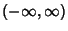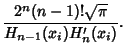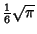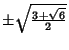Also called Hermite Quadrature. A Gaussian Quadrature over the intervalwith Weighting Function. The Abscissas for quadrature orderare given by the roots of the Hermite Polynomials, which occur symmetrically about 0. The Weights are(1)

whereis the Coefficient ofin. For Hermite Polynomials,(2)

so(3)(4)

so(5)

Using the Recurrence Relation(6)

yields(7)

and gives(8)

The error term is(9)

Beyer (1987) gives a table of Abscissas and weights up to=12.2 ± 0.707107 0.886227 3 0 1.18164 ± 1.22474 0.295409 4 ± 0.524648 0.804914 ± 1.65068 0.0813128 5 0 0.945309 ± 0.958572 0.393619 ± 2.02018 0.0199532

The Abscissas and weights can be computed analytically for small.23 04References

Beyer, W. H. CRC Standard Mathematical Tables, 28th ed. Boca Raton, FL: CRC Press, p. 464, 1987.

Hildebrand, F. B. Introduction to Numerical Analysis. New York: McGraw-Hill, pp. 327-330, 1956.